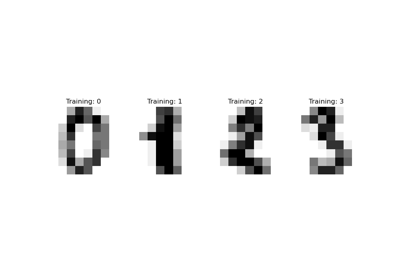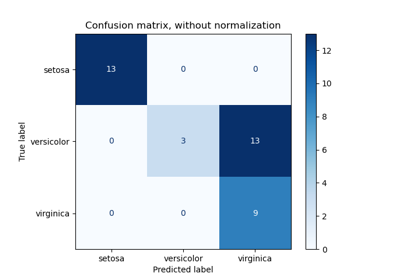# sklearn.metrics.plot_confusion_matrix¶

sklearn.metrics.plot_confusion_matrix(estimator, X, y_true, *, labels=None, sample_weight=None, normalize=None, display_labels=None, include_values=True, xticks_rotation='horizontal', values_format=None, cmap='viridis', ax=None)[source]

Plot Confusion Matrix.

Read more in the User Guide.

Parameters
estimatorestimator instance

Fitted classifier or a fitted Pipeline in which the last estimator is a classifier.

X{array-like, sparse matrix} of shape (n_samples, n_features)

Input values.

yarray-like of shape (n_samples,)

Target values.

labelsarray-like of shape (n_classes,), default=None

List of labels to index the matrix. This may be used to reorder or select a subset of labels. If None is given, those that appear at least once in y_true or y_pred are used in sorted order.

sample_weightarray-like of shape (n_samples,), default=None

Sample weights.

normalize{‘true’, ‘pred’, ‘all’}, default=None

Normalizes confusion matrix over the true (rows), predicted (columns) conditions or all the population. If None, confusion matrix will not be normalized.

display_labelsarray-like of shape (n_classes,), default=None

Target names used for plotting. By default, labels will be used if it is defined, otherwise the unique labels of y_true and y_pred will be used.

include_valuesbool, default=True

Includes values in confusion matrix.

xticks_rotation{‘vertical’, ‘horizontal’} or float, default=’horizontal’

Rotation of xtick labels.

values_formatstr, default=None

Format specification for values in confusion matrix. If None, the format specification is ‘d’ or ‘.2g’ whichever is shorter.

cmapstr or matplotlib Colormap, default=’viridis’

Colormap recognized by matplotlib.

axmatplotlib Axes, default=None

Axes object to plot on. If None, a new figure and axes is created.

Returns
displayConfusionMatrixDisplay

Examples

>>> import matplotlib.pyplot as plt  # doctest: +SKIP
>>> from sklearn.datasets import make_classification
>>> from sklearn.metrics import plot_confusion_matrix
>>> from sklearn.model_selection import train_test_split
>>> from sklearn.svm import SVC
>>> X, y = make_classification(random_state=0)
>>> X_train, X_test, y_train, y_test = train_test_split(
...         X, y, random_state=0)
>>> clf = SVC(random_state=0)
>>> clf.fit(X_train, y_train)
SVC(random_state=0)
>>> plot_confusion_matrix(clf, X_test, y_test)  # doctest: +SKIP
>>> plt.show()  # doctest: +SKIP


## Examples using sklearn.metrics.plot_confusion_matrix¶